# Inverse parabolic partial differential equation

An equation of the form(*)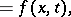where the formis positive definite. The variableplays the role of "inverse" time. The substitutionreduces equation (*) to the usual parabolic form. Parabolic equations of "mixed" type occur, for example,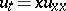is a direct parabolic equation forand an inverse parabolic equation for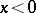, with degeneracy of the order for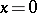.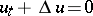(being the Laplace operator) see [a1].